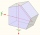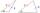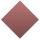# Sine - math problems

#### Number of problems found: 165

• RoadThe angle of a straight road is approximately 12 degrees. Determine the percentage of this road.
• RhombusInternal angles of rhombus is in ratio 2:3. How many times is the shorter diagonal longer than side of rhombus?
• Height differenceWhat height difference overcome if we pass road 1 km long with a pitch21 per mille?
• SteepleWe see the church tower from the road at an angle of 52°. When we zoom out to 29 meters away, it can be seen at an angle of 21°. How high is it?
• Inscribed and described circleFind the radii of a circle inscribed and circumscribed by a regular pentagon whose side measures 3 cm.
• The cable carThe cable car has a length of 3,5 kilometers and an angle of climb of 30 degrees. What is the altitude difference between the Upper and Lower stations?
• Two triangles SSATwo triangles can be formed with the given information. Use the Law of Sines to solve the triangles. A = 59°, a = 13, b = 14
• The staircaseThe staircase has a total height of 3.6 m and forms an angle of 26° with the horizontal. Calculate the length of the whole staircase.
• Hexagon 5The distance of parallel sides of regular hexagonal is 61 cm. Calculate the length of the radius of the circle described to this hexagon.
• Chord - TSThe radius of circle k measures 68 cm. Arc GH = 47 cm. What is TS?
• KitesBoys run kite on a cable of 68 meters long. What is the kite altitude, if the angle from the horizontal plane is 72°?
• An angleAn angle x is opposite side AB which is 10, and side AC is 15 which is hypotenuse side in triangle ABC. Calculate angle x.
• Equilateral triangleHow long should be the minimum radius of the circular plate to be cut equilateral triangle with side 19 cm from it?
• Largest angle of the triangleCalculate the largest angle of the triangle whose sides have the sizes: 2a, 3/2a, 3a
• Hexagon ACalculate area of regular hexagon inscribed in circle with radius r=9 cm.
• n-gonWhat is the side length of the regular 5-gon inscribed in a circle of radius 12 cm?
• Isosceles triangle 10In an isosceles triangle, the equal sides are 2/3 of the length of the base. Determine the measure of the base angles.
• DiagonalsRhombus has two diagonals e=14 dm and f=11 dm. Calculate the side angle and height of the rhombus.
• Triangle SAAThe triangle has one side long 71 m and its two internal angles is 60°. Calculate the perimeter and area of the triangle.
• DecagonCalculate the area and circumference of the regular decagon when its radius of a circle circumscribing is R = 1m

Do you have an interesting mathematical word problem that you can't solve it? Submit a math problem, and we can try to solve it.

We will send a solution to your e-mail address. Solved examples are also published here. Please enter the e-mail correctly and check whether you don't have a full mailbox.

Please do not submit problems from current active competitions such as Mathematical Olympiad, correspondence seminars etc...

Most natural application of trigonometry and trigonometric functions is a calculation of the triangles. Common and less common calculations of different types of triangles offers our triangle calculator. Word trigonometry comes from Greek and literally means triangle calculation.